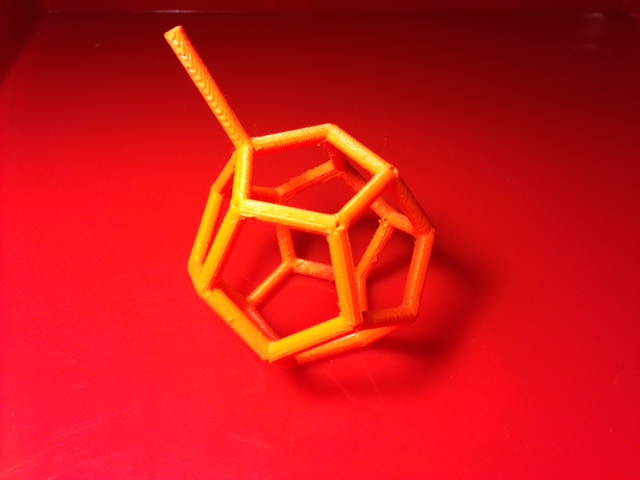# MakerHome Day 14 – Dodecabubbles## MakerHome Day 14 – Dodecabubbles

MakerHome Day 14 – Dodecabubbles 640 480 mathgrrl

Inspired by JMP’s Bubble Frames on Thingiverse, today we have a dodecahedron frame for dipping into bubble solution to make a minimal surface.

Settings: MakerWare “Low” with raft and supports, with a model at 80% of original size taking just over an hour.

Technical notes:  The vertex and edge data for the frame of the dodecahedron was taken from Mathematica using PolyhedronData[“Dodecahedron”, “Edges”]. Since I couldn’t figure out how to get a tubular wireframe directly, I cobbled the vertex and edge data together manually as shown below the fold, and then exported to .stl. Unfortunately this made a non-watertight model with ragged holes in the joins, which didn’t print correctly. Luckily all it took was using “Close Holes” in MeshLab to close up the model and make it suitable for printing. Both versions of the model looked the same in MakerWare, but Tinkercad could see the difference.

Stuff you might want to change:  The edges of the model are a bit too thick, so next time I print I’ll redesign them to be thinner.  In addition there are divots at each vertex that would be better if smoothed out.  In the long run it would be nice to see how five Platonic solids behave as bubble frames.

Mathematica code to generate the model:

```Graphics3D[{JoinForm["Round"],

Tube[{
{-Sqrt[1 + 2/Sqrt], 0, Root[1 - 20 #1^2 + 80 #1^4 &, 3]},
{-2 Sqrt[1 + 2/Sqrt], 0, 2 Root[1 - 20 #1^2 + 80 #1^4 &, 3]}}, 0.1],

Tube[{
{-Sqrt[1 + 2/Sqrt], 0, Root[1 - 20 #1^2 + 80 #1^4 &, 3]},
{Root[1 - 100 #1^2 + 80 #1^4 &, 1], 1/4 (-1 - Sqrt),Root[1 - 20 #1^2 + 80 #1^4 &, 2]},
{Root[1 - 20 #1^2 + 80 #1^4 &, 1], 1/4 (-3 - Sqrt), Root[1 - 20 #1^2 + 80 #1^4 &, 3]},
{Root[1 - 20 #1^2 + 80 #1^4 &, 2], 1/4 (-1 - Sqrt), Sqrt[5/8 + 11/(8 Sqrt)]},
{Sqrt[1/4 + 1/(2 Sqrt)], -(1/2), Sqrt[5/8 + 11/(8 Sqrt)]},
{Sqrt[5/8 + 11/(8 Sqrt)], 1/4 (-1 - Sqrt), Root[1 - 20 #1^2 + 80 #1^4 &, 3]},
{Sqrt[1 + 2/Sqrt], 0, Root[1 - 20 #1^2 + 80 #1^4 &, 2]},
{Sqrt[5/8 + 11/(8 Sqrt)], 1/4 (1 + Sqrt), Root[1 - 20 #1^2 + 80 #1^4 &, 3]},
{Sqrt[1/4 + 1/(2 Sqrt)], 1/2, Sqrt[5/8 + 11/(8 Sqrt)]},
{Root[1 - 20 #1^2 + 80 #1^4 &, 2], 1/4 (1 + Sqrt), Sqrt[5/8 + 11/(8 Sqrt)]},
{Root[1 - 20 #1^2 + 80 #1^4 &, 1], 1/4 (3 + Sqrt), Root[1 - 20 #1^2 + 80 #1^4 &, 3]},
{Root[1 - 100 #1^2 + 80 #1^4 &, 1], 1/4 (1 + Sqrt), Root[1 - 20 #1^2 + 80 #1^4 &, 2]},
{-(1/2) Sqrt[1 + 2/Sqrt], 1/2, Root[1 - 100 #1^2 + 80 #1^4 &, 1]},
{-(1/2) Sqrt[1 + 2/Sqrt], -(1/2), Root[1 - 100 #1^2 + 80 #1^4 &, 1]},
{Root[1 - 100 #1^2 + 80 #1^4 &, 1], 1/4 (-1 - Sqrt), Root[1 - 20 #1^2 + 80 #1^4 &, 2]}}, 0.1],

Tube[{
{-Sqrt[1 + 2/Sqrt], 0, Root[1 - 20 #1^2 + 80 #1^4 &, 3]},
{Root[1 - 100 #1^2 + 80 #1^4 &, 1], 1/4 (1 + Sqrt), Root[1 - 20 #1^2 + 80 #1^4 &, 2]}}, 0.1],

Tube[{
{-Sqrt[1 + 2/Sqrt], 0, Root[1 - 20 #1^2 + 80 #1^4 &, 3]},
{Root[1 - 5 #1^2 + 5 #1^4 &, 1], 0, Sqrt[5/8 + 11/(8 Sqrt)]},
{Root[1 - 20 #1^2 + 80 #1^4 &, 2], 1/4 (-1 - Sqrt), Sqrt[5/8 + 11/(8 Sqrt)]}}, 0.1],

Tube[{
{Sqrt[1 + 2/Sqrt], 0, Root[1 - 20 #1^2 + 80 #1^4 &, 2]},
{Sqrt[1/10 (5 + Sqrt)], 0, Root[1 - 100 #1^2 + 80 #1^4 &, 1]},
{Root[1 - 20 #1^2 + 80 #1^4 &, 3], 1/4 (-1 - Sqrt), Root[1 - 100 #1^2 + 80 #1^4 &, 1]},
{Sqrt[1/8 + 1/(8 Sqrt)], 1/4 (-3 - Sqrt), Root[1 - 20 #1^2 + 80 #1^4 &, 2]},
{Sqrt[5/8 + 11/(8 Sqrt)], 1/4 (-1 - Sqrt), Root[1 - 20 #1^2 + 80 #1^4 &, 3]}}, 0.1],

Tube[{
{Root[1 - 20 #1^2 + 80 #1^4 &, 1], 1/4 (-3 - Sqrt), Root[1 - 20 #1^2 + 80 #1^4 &, 3]},
{Sqrt[1/8 + 1/(8 Sqrt)], 1/4 (-3 - Sqrt), Root[1 - 20 #1^2 + 80 #1^4 &, 2]}}, 0.1],

Tube[{
{Root[1 - 20 #1^2 + 80 #1^4 &, 1], 1/4 (3 + Sqrt), Root[1 - 20 #1^2 + 80 #1^4 &, 3]},
{Sqrt[1/8 + 1/(8 Sqrt)], 1/4 (3 + Sqrt), Root[1 - 20 #1^2 + 80 #1^4 &, 2]},
{Root[1 - 20 #1^2 + 80 #1^4 &, 3], 1/4 (1 + Sqrt), Root[1 - 100 #1^2 + 80 #1^4 &, 1]},
{-(1/2) Sqrt[1 + 2/Sqrt], 1/2, Root[1 - 100 #1^2 + 80 #1^4 &, 1]}}, 0.1],

Tube[{
{Sqrt[5/8 + 11/(8 Sqrt)], 1/4 (1 + Sqrt), Root[1 - 20 #1^2 + 80 #1^4 &, 3]},
{Sqrt[1/8 + 1/(8 Sqrt)], 1/4 (3 + Sqrt), Root[1 - 20 #1^2 + 80 #1^4 &, 2]}}, 0.1],

Tube[{
{Root[1 - 20 #1^2 + 80 #1^4 &, 2], 1/4 (1 + Sqrt), Sqrt[5/8 + 11/(8 Sqrt)]},
{Root[1 - 5 #1^2 + 5 #1^4 &, 1], 0, Sqrt[5/8 + 11/(8 Sqrt)]}}, 0.1],

Tube[{
{-(1/2) Sqrt[1 + 2/Sqrt], -(1/2), Root[1 - 100 #1^2 + 80 #1^4 &, 1]},
{Root[1 - 20 #1^2 + 80 #1^4 &, 3], 1/4 (-1 - Sqrt), Root[1 - 100 #1^2 + 80 #1^4 &, 1]}}, 0.1],

Tube[{
{Sqrt[1/4 + 1/(2 Sqrt)], -(1/2), Sqrt[5/8 + 11/(8 Sqrt)]},
{Sqrt[1/4 + 1/(2 Sqrt)], 1/2, Sqrt[5/8 + 11/(8 Sqrt)]}}, 0.1],

Tube[{
{Sqrt[1/10 (5 + Sqrt)], 0, Root[1 - 100 #1^2 + 80 #1^4 &, 1]},
{Root[1 - 20 #1^2 + 80 #1^4 &, 3], 1/4 (1 + Sqrt), Root[1 - 100 #1^2 + 80 #1^4 &, 1]}}, 0.1]
}]```

——————

As an Amazon Associate we earn from qualifying purchases, so if you’ve got something you need to pick up anyway, going to Amazon through this linkwill help us keep Hacktastic running. Thanks! :)

Share